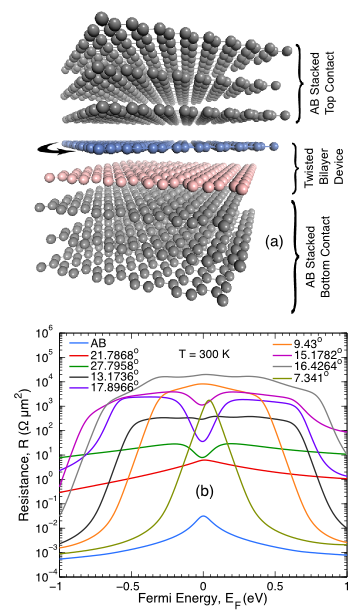# Misoriented Layers of Graphene

There is rapidly growing interest in vertically stacked van der Waals materials for electronic device applications. In such structures the interfaces between different materials will, in general, be misoriented with respect to each other. THz cutoff frequencies have been predicted for such devices. Understanding the effect of the misorientation on the interlayer resistance is required to fully understand the design requirements and performance of proposed vertically stacked devices.

The most well studied and well understood of the van der Waals material are graphite and graphene. The effect of misorientation on the electronic structure of bilayer graphene has been studied extensively both theoretically and experimentally. After a few degrees misorientation, the in-plane dispersion becomes linear, and after about 10 degrees misorientation, the in-plane velocity is the same as that of single-layer graphene. Thus, the two misorineted layers of graphene act as if they are electronically decoupled.Rotated interface of AB stacked graphite: (a) Atomistic geometry and (b) room temperature coherent contact resistance of twisted bilayer graphene as a function of Fermi energy for different rotation angles.

The calculated coherent interlayer resistance as a function of rotation angle θ is found to vary by 16 orders of magnitude as the misorientation angle changes from zero to 30 degrees. The values vary from approximately 1015 Ωμm2 to 0.1 Ωμm2. The room-temperature, phonon-mediated inter-layer resistance of misoriented bilayer graphene shows far less dependence on the misorientation angle. It changes by less than an order of magnitude as the angle varies from zero to 30 degrees. is approximately 100 Ωμm2 over a range of intermediate rotation angles. Experimental measurements found approximately an order of magnitude larger resistance that varied from 750 Ωμm2 to 3400 Ωμm2 as the angle varied from 5o to 24o.

We determined the coherent, interlayer resistance of a misoriented, rotated interface between two stacks of AB graphite for a variety of misorientation angles. The quantum-resistance of the ideal AB stack is on the order of 1 to 10 mΩμm2. For small rotation angles, the coherent interlayer resistance exponentially approaches the ideal quantum resistance at energies away from the charge neutrality point. Over a range of intermediate angles, the resistance increases exponentially with cell size for minimum size unit cells. Larger cell sizes, of similar angles, may not follow this trend. The energy dependence of the interlayer transmission is described.